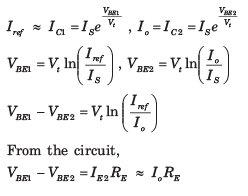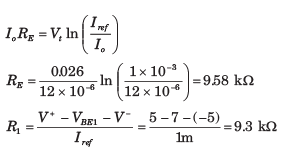Courses

# Basic BJT Circuits - MCQ Test

## 20 Questions MCQ Test GATE ECE (Electronics) 2022 Mock Test Series | Basic BJT Circuits - MCQ Test

Description
This mock test of Basic BJT Circuits - MCQ Test for Electronics and Communication Engineering (ECE) helps you for every Electronics and Communication Engineering (ECE) entrance exam. This contains 20 Multiple Choice Questions for Electronics and Communication Engineering (ECE) Basic BJT Circuits - MCQ Test (mcq) to study with solutions a complete question bank. The solved questions answers in this Basic BJT Circuits - MCQ Test quiz give you a good mix of easy questions and tough questions. Electronics and Communication Engineering (ECE) students definitely take this Basic BJT Circuits - MCQ Test exercise for a better result in the exam. You can find other Basic BJT Circuits - MCQ Test extra questions, long questions & short questions for Electronics and Communication Engineering (ECE) on EduRev as well by searching above.
QUESTION: 1

Solution:
QUESTION: 2

### For the transistor in circuit shown in fig. β = 200. Determine the value of VC for given value of VB in question.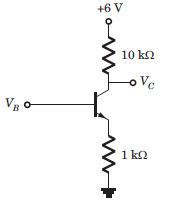If VB = 1V

Solution: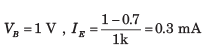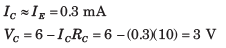QUESTION: 3

### For the transistor in circuit shown in fig. β = 200. Determine the value of IE and IC for given value of VB in question.If VB = 2V

Solution: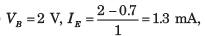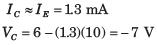Transistor is in stauration. The saturation voltage
VCE = 0.2V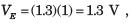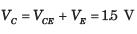QUESTION: 4

The transistor in circuit shown in fig. has β = 200. Determine the value of voltage Vo for given value of VBB.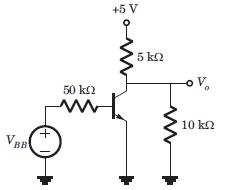If VBB = 0

Solution:

VBB = 0, Transistor is in cutoff region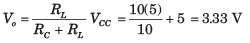QUESTION: 5

The transistor in circuit shown in fig. has β = 200. Determine the value of voltage Vo for given value of VBB.If VBB = 1V

Solution: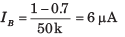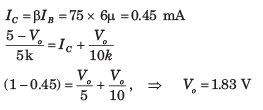QUESTION: 6

The transistor in circuit shown in fig. has β = 200. Determine the value of voltage Vo for given value of VBB.If VBB = 2V

Solution: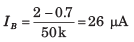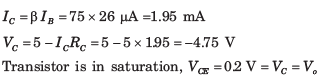QUESTION: 7

The transistor shown in the circuit of fig. has β = 150. Determine Vo for given value of
IQ in question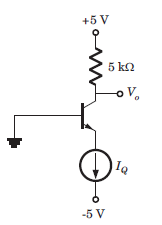If IQ = 0.1 mA

Solution:

I= 0.1 mA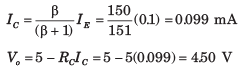QUESTION: 8

The transistor shown in the circuit of fig. has β = 150. Determine Vo for given value of  IQ in questionIf IQ = 0.5 mA

Solution:

IE = IQ = 0.5 mA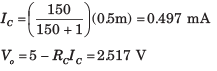QUESTION: 9

The transistor shown in the circuit of fig. has β = 150. Determine Vo for given value of  IQ in questionIf IQ = 2mA

Solution:

Transistor is in saturation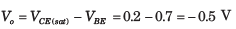QUESTION: 10

For the circuit in fig. VB = V and  β = 50. The value of VB is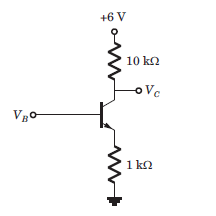Solution: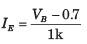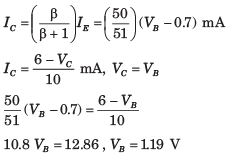QUESTION: 11

For the circuit shown in fig. VCB = 0.5 V and β =100. The value of IQ is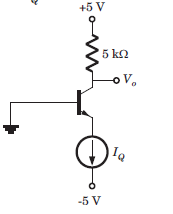Solution: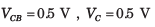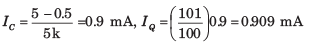QUESTION: 12

For the circuit shown in fig. the emitter voltage is VE = 2 V. The value of α is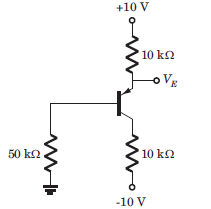Solution: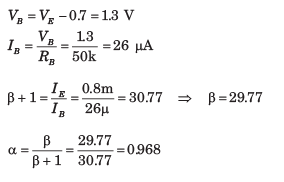QUESTION: 13

For the transistor in fig. β = 50. The value of voltage VEC  is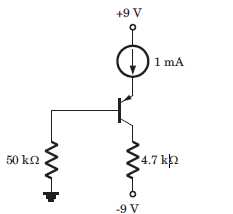Solution: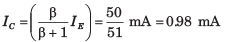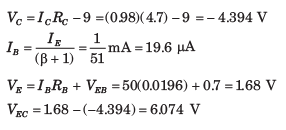QUESTION: 14

The current gain of the transistor shown in the circuit of fig. is β = 125. The Q-point values (ICQ , VCEQ ) are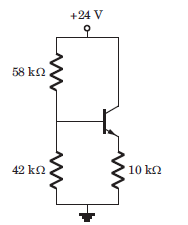Solution: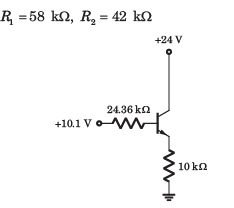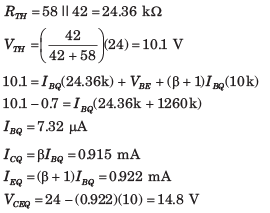QUESTION: 15

For the circuit shown in fig. , let β = 75. The Q-point (ICQ , VCEQ ) is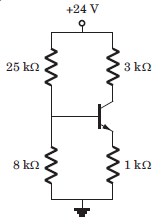Solution: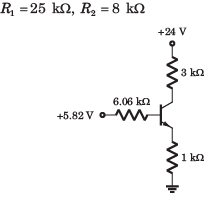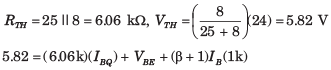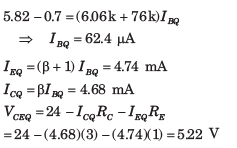QUESTION: 16

The current gain of the transistor shown in the circuit of fig. is β = 100. The values of Q-point (ICQ , VCEQ ) is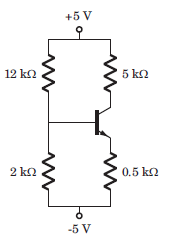Solution:

R1 =12 Ω, R2 = 2 Ω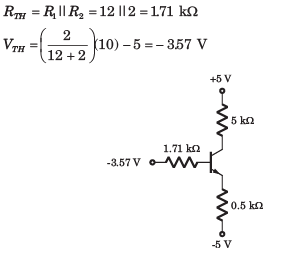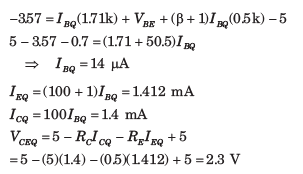QUESTION: 17

For the circuit in fig. , let β = 60. The value of VECQ  is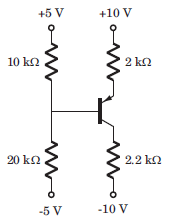Solution: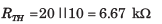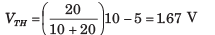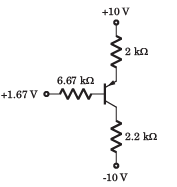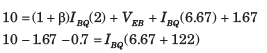QUESTION: 18

All transistor in the N output mirror in fig. are matched with a finite gain β and early voltage VA = ∞. The expression for each load current is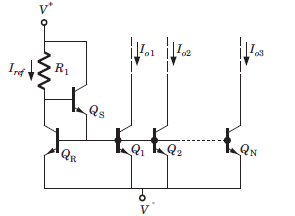Solution: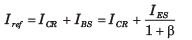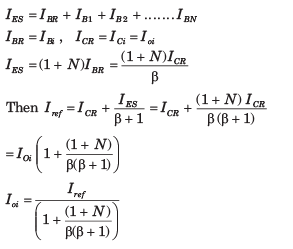QUESTION: 19

Consider the basic three transistor current source in fig. Assume all transistor are matched with finite gain and early voltage VA  = ∞.The expression for Io is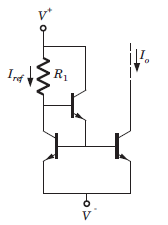Solution: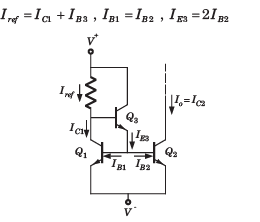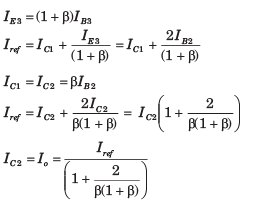QUESTION: 20

Consider the wilder current source of fig. Both of transistor are identical and β>>1 and VBE1 = 0.7 V. The value of resistance R1 and RE to produce Iref  = 1 mA and Io = 12 μA is (Vt = 0.026)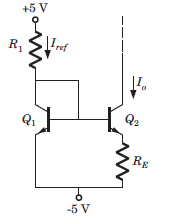Solution:

If β>>1 and transistor are identical